Yes, I knowOf bits and bytes

In computer science, we often express data size using fancy words like bit, bytes or words. But what do they represent exactly? And was it always like that?

Share

At the beginning, there was the bit

Well …​ maybe not at the very beginning. In the early days of computing, it was uncertain how large should be the most elementary "bit" (pun intended) of information a computer could handle.

Some early designs used trits, a tri-state information building blocks. That choice was not made at random, but because ternary numbers have the lowest radix economy and thus allowed a more efficient representation of numbers (remember, at that time memory was very sparse!)

Computers based on trits used either balanced ternary systems (where one trit could hold the value -1, 0, or +1) or unbalanced ternary systems (0, 1, 2). Some designs were also based on a tri-state true/false/undefined arithmetic. While not very successful in computer design, a close concept, the three-state logic, which adds an high-impedance state in addition to the high and low logic levels, is widely used in digital electronic engineering.

Occasionally, research papers are still published to remind us the advantages of ternary designs. However, the overwhelming majority of computers today are based on binary numbers.

In a binary computer, the most basic unit of information is the bit. It stands for "binary digit" and, as its name implies, a bit can only take two different values. By convention, we call them 1 and 0, true and false, high and low, or, in formal logic, ⊤ and . Those are just conventions though. We could call them the rectangle and triangle states, the blue and green ones, or the hot and the cold.

In fact, at the hardware level, any physical properties can be used to hold a bit, as long as we can distinguish two different states. Thus, as a non-exhaustive list, we can represent a bit:

• as a "hole" or "no-hole" in electro-mechanical systems like punch cards when used in binary mode;

• as light/no-light in an optical system;

• as a positive/negative polarity on a magnetic storage media;

• as different voltages (close to 0V for false, close to +5V for true — or the other way around) in an electronic system;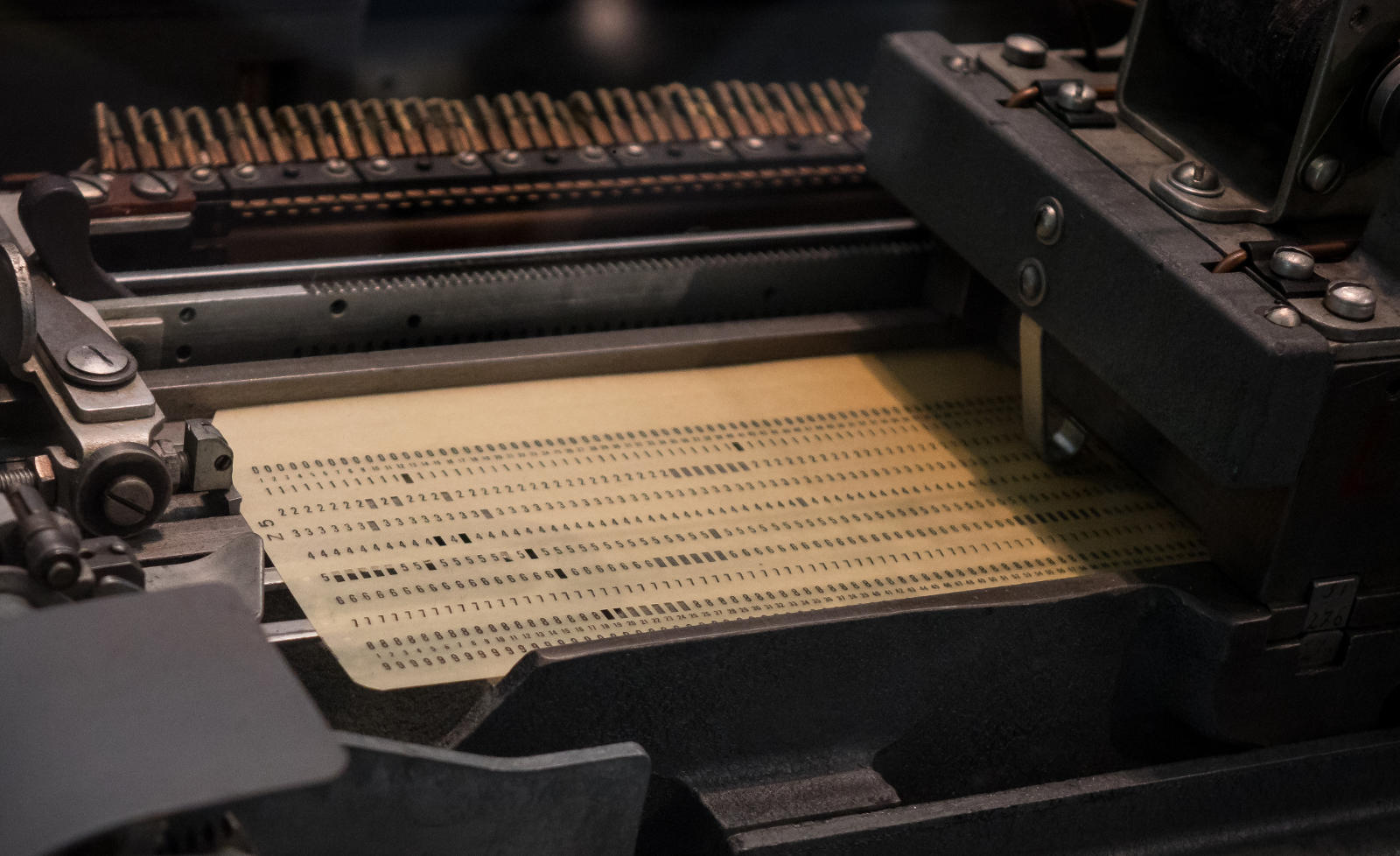Some branches of the mathematics extensively studied the binary operations. The most well-known are the boolean algebra and the binary arithmetic.

The boolean algebra considers binary values as truth values and deals mainly with logical operators like the conjunction and, the disjunction or, and the negation not.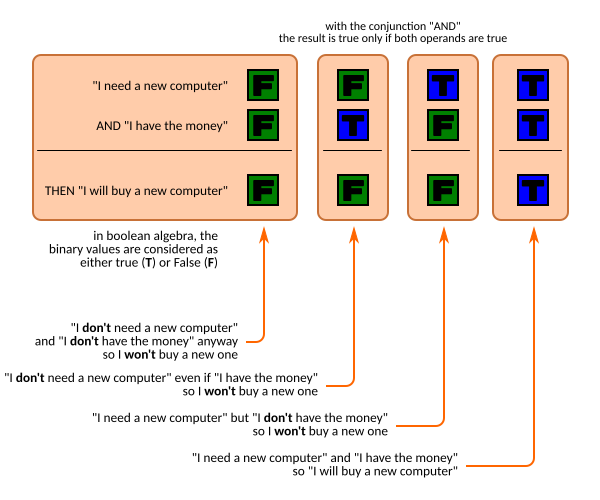The binary arithmetic considers binary values as the digits 0 and 1 and defines the addition, subtraction, multiplication, and division for binary numbers.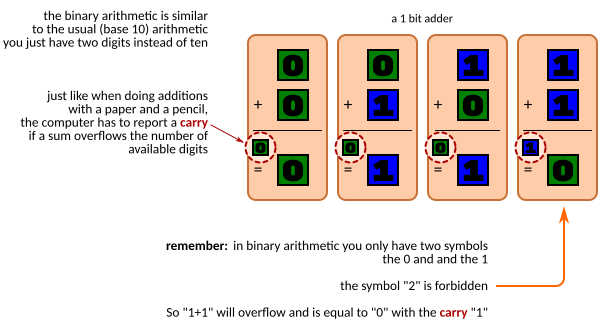When you have one bit, you have only two different states at your disposition. This is very little to represent real-life data.

However, the bits can be combined to represent larger values:

• With one bit, we can represent two values.
Let’s call them 0 and 1.

• With two bits, we have 2✕2 values.
Say 0, 1, 2 and 3.

• With three bits, we have 2✕2✕2 values.
0, 1, 2, 3, 4, 5, 6 and 7.

• And with each extra bit, we double the number of possible values.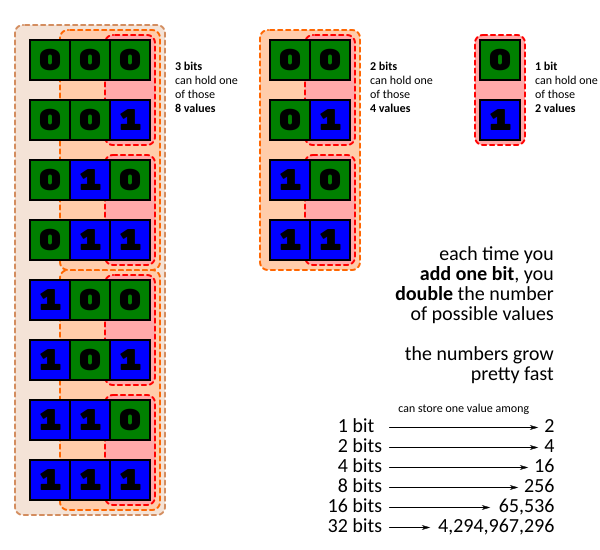As an exercise to test your comprehension, try to find the minimum number of bits required to store the following information:

Minimum number of bits required to store some real-world data
Information Number of bits required

The state of a light bulb (on/off)

The day of the week (Monday to Sunday)

The age of a person (assuming a max of 120 years)

The number of people on earth

That byte might bite you

In French, we have the word "octet" that unambiguously define a group of 8 bits. Actually, this word exists in English too, but more often we encounter the word byte instead. Today a byte is almost always used to represent a group of 8 bits, but in early computing days, it was used to represent a "small group of bits," and it could as well represent a group of 4, 6 or 7 bits.

It was only with the emergence of 8-bits microprocessors in the 70s the byte was informally defined as an 8 bits quantity. Around the same time, the less popular word nibble was introduced to represent a 4-bit value (that is, half of a "modern" byte).

So with 8 bits, a byte can hold 2✕2✕2✕2✕2✕2✕2✕2 values, That is 2⁸ or 256 different values. If we conventionally start representing values with 0, that allows counting up to 255.

I let you do the necessary calculations to know how many different values can be stored in a 4-bits nibble (hint: despite a nibble being half of the size of a byte, the answer is not 128).

 A bit can hold 2 values A nibble can hold A byte can hold 256 values

A word on words

A word is corresponding to the amount of data a microprocessor can handle in one operation.

• 8 bits microprocessors use 8 bits words (1 byte).

• 16 bits microprocessors use 16 bits words (2 bytes)

• 32 bits microprocessors use 32 bits words (4 bytes)

• 64 bits microprocessors use 64 bits words (8 bytes)

Note: More formally, the microprocessor "size" corresponds usually to the size of the internal data registers of the microprocessors.

It does not mean a n-bit microprocessor is limited to handle n bits data, but if it needs to handle data requiring more than n bits to represent them, it will have to do it in several operations instead of just one.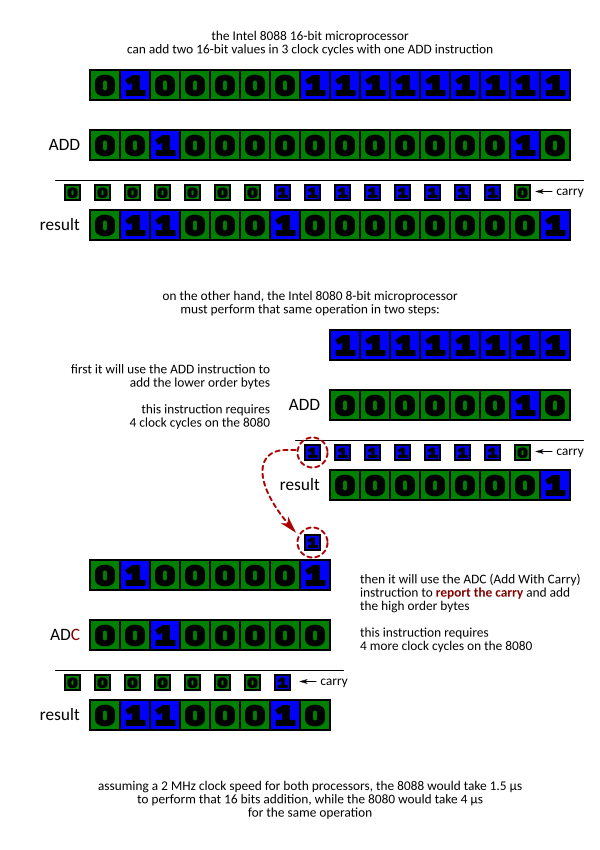The microprocessor word size also has an impact on the speed it can load and store data since, while not mandatory, the data bus width is often corresponding to the CPU word size.

Let’s consider a very simple computer made of some amount of RAM, a microprocessor, and a video card. Imagine now we want to process and display a 16kB (16000 bytes) picture stored in RAM. It means the microprocessor will have to read the data from the RAM, somehow process them (say, converting the color image to grayscale) and finally copy the modified data to the video card frame buffer.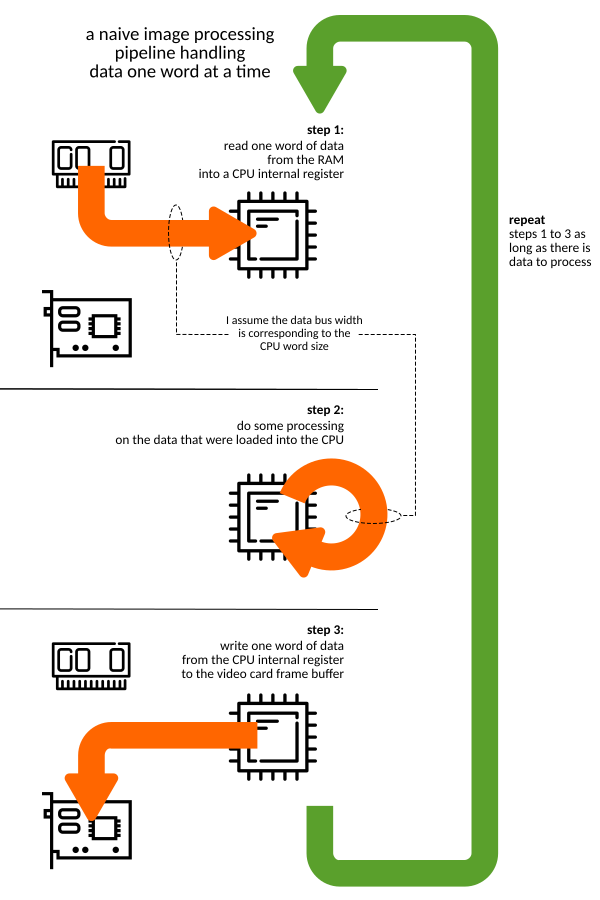In a naive implementation, the microprocessor will read, process, then write the data one word at a time. So, an 8-bits microprocessor will have to perform 16000 read, and 16000 write operations to copy the image.

On the other hand, a 16 bits microprocessor handle words of 2 bytes at a time. So it would need only 8000 read and 8000 write operations to move the same amount of data.

Once again, I let you make the calculations for the other common word sizes:

Number of operations required to move 16000 bytes of data
Data bus size # of operations

8-bit data bus (moves 1 byte at a time)

16000 write

16-bit data bus (moves 2 bytes at a time)

8000 write

32-bit data bus (moves 4 bytes at a time)

64-bit data bus (moves …​ bytes at a time)

Summary

I hope this article helped you clarifying some fundamental units we use a lot in computer science. Remember: one bit can hold one of two possible values. Always. A byte is eight bits, most of the time. And a word, well, it depends.

If you enjoyed that article, you might also be interested in my infographic The Microprocessor Familly Tree which shows the twisted path the industry followed from the early 4-bits microprocessors of the 70s to the powerful processors we know today. And let me know on Twitter or Facebook if you want more articles like this one!